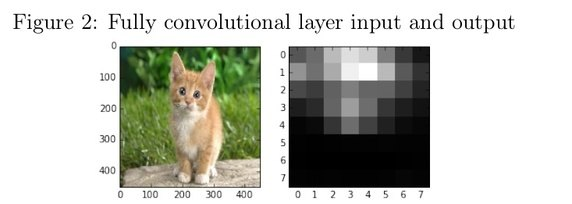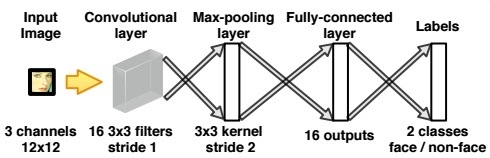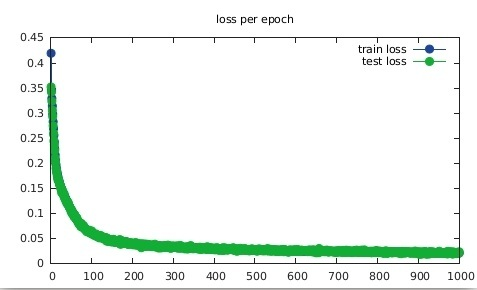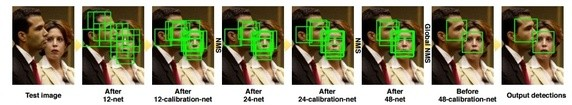In this post we’re going to build a super fast face detector!

Project on GitHub: IggyShone/fast_face_detector

Face detection, a sub problem of object detection , is a challenging task due to the fact that faces may appear in various pose, scale, facial expression, occlusion, and lighting settings. One of the simplest and common ways to perform an object detection in the world of deep neural networks is a sliding window approach where an image is scanned sequentially, by running a network on it, inspecting each fixed size window at a time. Unfortunately, despite giving (if trained correctly) promising accuracy ,this routine is costly and therefore greatly degenerates a given detector speed. A better solution would be to transform a given network into a fully-convolutional network by converting fully-connected layers to convolution layers. The reason therefore lay in the fact that convolutional outputs are shared between multiple windows.

The output of a fully convolutional network (FCN) on a given image is a probability map for the existence of the object in each window. The size of the window is the network input size while the stride between each window is decided by the amount of down sampling the network performs.

For instance: consider activating a fully convolutional network with input size of 227 and stride of 32 over an input image of size 451 × 451. The equation for output map:

output = (input − network_input_size)/stride + 1 = (451 − 227)/32 + 1 = 8Each cell (i, j) in the heat-map contains the probability for an object (a face in our case) to exist in a window with bottom left point (32i, 32j) of spatial size 32 × 32.
For more detail explanation on convolutional neural networks and FCN in general and the transformation between those two in detail please refer to: CS231n Convolutional Neural Networks for Visual Recognition

Step 1: face / non-face classifier

When inspecting a specific window at some size we’d need to binary classify it deciding weather the pixels in that window constitutes a face or not.

A simple shallow convolutional network would do the job. The network receives as input a patch of size 12 × 12 from a RGB image - and classifies it as either face or non-face. As positive samples we’d use AFLW faces while for the negative we’d crop random patches from the PASCAL data-set

System SchemeModel
``````local model = nn.Sequential();
-- input 3x12x12
-- outputs 16x10x10
-- outputs 16x4x4
-- outputs 16x1x1
-- outputs 2x1x1
```
```

Definitions
``````----------------
-- LOSS FUNCTION
----------------
local criterion = nn.CrossEntropyCriterion()

---------------
-- TRAINING
---------------

------------------------------
-- Closure with mini-batches
------------------------------
local counter = 0
local feval = function(x)
if x ~= parameters then
parameters:copy(x)
end
local start_index = counter * opt.batch_size + 1
local end_index = math.min(opt.train_size, (counter + 1) *
opt.batch_size)
if end_index == opt.train_size then
counter = 0
else
counter = counter + 1
end
local batch_inputs = data[{{start_index, end_index}, {}}]
local batch_targets = labels[{{start_index, end_index}}]

-- 1. compute outputs (log probabilities) for data point
local batch_outputs = model:forward(batch_inputs)
-- 2. compute the loss of these outputs, measured against
the true labels in batch_target
local batch_loss = criterion:forward(batch_outputs,
batch_targets)
-- 3. compute the derivative of the loss wrt the outputs of
the model
local loss_doutput = criterion:backward(batch_outputs,
batch_targets)
-- 4. use gradients to update weights
model:backward(batch_inputs, loss_doutput)

end
```
```

Training is done on mini-batches while minimizing a cross entropy loss function.

Optimization
``````-- OPTIMIZE
-----------
local train_losses = {}
local test_losses = {}

-- # epoch tracker
epoch = epoch or 1

for i = 1,opt.epochs do

trimModel(model)

-- shuffle at each epoch
local shuffled_indexes =
torch.randperm(data:size(1)):long()
data = data:index(1,shuffled_indexes)
labels = labels:index(1,shuffled_indexes)

local train_loss_per_epoch = 0
-- do one epoch
print('==> doing epoch on training data:')
print("==> online epoch # " .. epoch .. ' [batchSize = ' ..
opt.batch_size .. ']')

for t = 1,opt.train_size,opt.batch_size do
if opt.optimization == 'sgd' then
_, minibatch_loss  = optim.sgd(feval, parameters,
optimState)
print('mini_loss: '..minibatch_loss)
train_loss_per_epoch = train_loss_per_epoch +
minibatch_loss
end
end
-- update train_losses average among all the mini batches
train_losses[#train_losses + 1] = train_loss_per_epoch /
(math.ceil(opt.train_size/opt.batch_size)-1)

-------------
-- TEST
-------------
trimModel(model)

local test_data = data[{{opt.train_size+1, data:size(1)},
{}}]
local test_labels = labels[{{opt.train_size+1,
data:size(1)}}]

local output_test = model:forward(test_data)
local err = criterion:forward(output_test, test_labels)

test_losses[#test_losses + 1] = err
print('test error ' .. err)

test_losses[#test_losses]}

end
```
```

We plot the cost function value for test and train after each epoch and monitor the classification error. Also compactly saving the model at end.
``````
--------------------------------------------------------
--  PLOTTING TESTING/TRAINING LOSS/CLASSIFICATION ERRORS
--------------------------------------------------------
gnuplot.pdffigure('loss_12net.pdf')
gnuplot.plot({'train loss',torch.range(1, #train_losses),torch.Tensor(train_losses)},
{'test loss',torch.Tensor(test_losses)})
gnuplot.title('loss per epoch')
gnuplot.figure()

---------------
-- SAVING MODEL
---------------
local fmodel = model : clone (): float ()
for i =1 ,# fmodel.modules do
local layer = fmodel : get ( i )
if layer.output ~= nil then
layer.output = layer.output.new ()
end
end
end

torch.save('model_12net.net', fmodel)
```
```

ResultsWe see that generalization succeeded (blue curve is hidden by the green one) with a classification error of 0.0145836.

Step 2: Repeat step 1 only this time with 24×24 and 48×48 sized windows.

The difference from the previous step is the source of the negative labels. For the 24×24 net, each window classified as positive in the 12×12 net would now serve as a negative example. This method is known as negative boostraping. Apply same to the 48×48 net w.r.t 24×24.
• So far We have built three stand-alone convolutional networks which constitutes the main building blocks of the face detector we just about to describe its pipeline.

Step 3: Detector pipeline
Given a test image, we’d want quickly to perform a dense scan to reject as much as possible of the detection windows though maintaining a high recall (eliminate many false negatives but keep many true positives). The 12×12 net is fast in that sense due to its relatively shallowness.
There is a problem however. Practically a face in an image could come up with different sizes (not necessarily fit to the fixed convolution nets input size). Thus we must scan the image across different scales. In computer vision this is called an image pyramid.If the face size is X, the test image is first built into image pyramid to cover faces at different scales and each level in the image pyramid is re-sized by 12X as the input image for the 12×12 net.

Creating image pyramid
``````local smallestImgDim = 100000
scales = {} -- list of scales
-- 20 is a system hyper param dependable on our prior knowledge
-- on face sizes. Acurracy vs performance tradeoff.
for k =1 ,20 do
local scale = 12/( smallestImgDim /20+ smallestImgDim *(k
-1)/20)
if scale * smallestImgDim < 12 then break end
table.insert (scales , scale )
end

-- create pyramid packer and unpacker. scales is a table with
-- all the scales you with to check.
local unpacker = nn.PyramidUnPacker(model)
local packer = nn.PyramidPacker(model, scales)
```
```
Therefore the result of that dense scan across different scales (via the 12×12 net) is a map of confidence scores for faces detections.
``````
-- dense scan across different scales
local multiscale  = model_12net:forward(pyramid)
-- unpack pyramid , distributions will be table of tensors
local distributions = unpacker_12:forward(multiscale ,
coordinates)
local val, ind, res = 0
local detections_12net = {}

-- iterate on the map of confidence scores
-- determining a face if p>threshold
for j = 1,#distributions do
local boxes = {}
distributions[j]:apply(math.exp)
vals, ind = torch.max(distributions[j],1)
ind_data = torch.data(ind)
--collect pos candidates (with threshold p>0.5)
local size = vals:size(2)
for t = 1,ind:nElement()-1 do
x_map = math.max(t%size,1)
y_map = math.ceil(t/size)
--converting to orig. sample coordinate
x = math.max((x_map-1)*2 ,1)
y = math.max((y_map-1)*2 ,1)

if ind[y_map][x_map] == 1 then
--prob. for a face
table.insert(boxes, {x,y,x+11,y+11,vals
[y_map][x_map]})
end
end
```
```

A side effect due to the many small windows involved could be an overflow of positive overlapped detection. Non-maximum suppression (NMS) is applied to eliminate highly overlapped detection windows.
``````
local pos_suspects_boxes = torch.Tensor(boxes)
local nms_chosen_suspects = nms(pos_suspects_boxes, 0.5)

if #nms_chosen_suspects:size() ~= 0 then
pos_suspects_boxes =
pos_suspects_boxes:index(1,nms_chosen_suspects)
```
```

The remaining detection windows are cropped out and resized into 24×24 images.
``````for p = 1,pos_suspects_boxes:size(1) do
--scalling up suspected box to orig size image
sus = torch.div(pos_suspects_boxes[p],scales[j])
sus:apply(math.floor)
croppedDetection = image.crop(img, sus,
sus, sus, sus)
croppedDetection = image.scale(croppedDetection,
24, 24)
table.insert(detections_12net,
{croppedDetection:resize(1,3,24,24), sus,
sus, sus, sus})
end
end
end
```
```

Then serve as input images for the 24×24 to further reject many of the remaining detection windows.
``````---- Use 24 net to run on each 12 net detection ----
local detections_24net = {}
for d = 1,#detections_12net do
local dist = model_24net:forward(detections_12net[d])
if math.exp(dist) > math.exp(dist
) then
--image.scale(croppedDetection, 24, 24)
table.insert(detections_24net,{detections_12net[d]
, detections_12net[d],
detections_12net[d], detections_12net[d]
, math.exp(dist)})
end
end
```
```
Same as before, the positive detection windows are applied with NMS to further reduce the number of detection windows.
``````if #detections_24net ~= 0 then
local pos_suspects_boxes =
torch.Tensor(detections_24net)
local nms_chosen_suspects = nms(pos_suspects_boxes, 0.5)

if #nms_chosen_suspects:size() ~= 0 then
pos_suspects_boxes =
pos_suspects_boxes:index(1,nms_chosen_suspects)
end

for n=1, pos_suspects_boxes:size(1) do
sus = pos_suspects_boxes[n]
table.insert(detections,sus)
end
end
```
```
Now repeat Step 3 but change everywhere from 12×12 to 24×24 and 24×24 to 48×48.

The overall process could be depicted as:Evaluation

To evaluate the detector we’d use the Face Detection Data Set and Benchmark. We’d need to save the detections in the elliptical format described here.
``````--find circles (simple ellipse) enclosing
--each bounding box and report detections
for d = 1,#detections do
box = detections[d]
box),2)+math.pow((box - box),2))
centerX = box + math.floor((box - box)/2)
centerY = box + math.floor((box - box)/2)
-- write detectiodetections_24netn in ellipse format